# Flip-flop (electronics)

Flip-flop (electronics)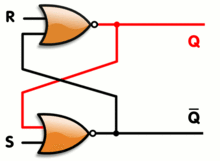An SR latch, constructed from a pair of cross-coupled NOR gates. Red and black mean logical '1' and '0', respectively.

In electronics, a flip-flop or latch is a circuit that has two stable states and can be used to store state information. The circuit can be made to change state by signals applied to one or more control inputs and will have one or two outputs. It is the basic storage element in sequential logic. Flip-flops and latches are a fundamental building block of digital electronics systems used in computers, communications, and many other types of systems.

Flip-flops and latches are used as data storage elements. Such data storage can be used for storage of state, and such a circuit is described as sequential logic. When used in a finite-state machine, the output and next state depend not only on its current input, but also on its current state (and hence, previous inputs.) It can also be used for counting of pulses, and for synchronizing variably-timed input signals to some reference timing signal.

Flip-flops can be either simple (transparent or opaque) or clocked (synchronous or edge-triggered); the simple ones are commonly called latches. The word latch is mainly used for storage elements, while clocked devices are described as flip-flops.

## History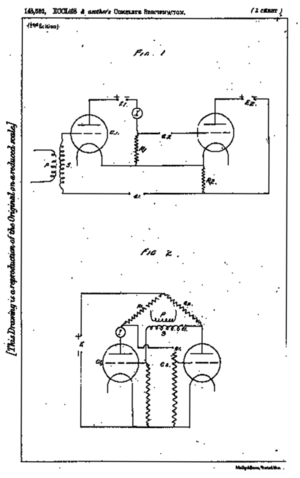Flip-flop schematics from the Eccles and Jordan patent filed 1918, one drawn as a cascade of amplifiers with a positive feedback path, and the other as a symmetric cross-coupled pair

The first electronic flip-flop was invented in 1918 by William Eccles and F. W. Jordan. It was initially called the Eccles–Jordan trigger circuit and consisted of two active elements (vacuum tubes). Such circuits and their transistorized versions were common in computers even after the introduction of integrated circuits, though flip-flops made from logic gates are also common now.

Early flip-flops were known variously as trigger circuits or multivibrators. A multivibrator is a two-state circuit; they come in several varieties, based on whether each state is stable or not: an astable multivibrator is not stable in either state, so it acts as a relaxation oscillator; a monostable multivibrator makes a pulse while in the unstable state, then returns to the stable state, and is known as a one-shot; a bistable multivibrator has two stable states, and this is the one usually known as a flip-flop. However, this terminology has been somewhat variable, historically. For example:

• 1942 – multivibrator implies astable: "The multivibrator circuit (Fig. 7-6) is somewhat similar to the flip-flop circuit, but the coupling from the anode of one valve to the grid of the other is by a condenser only, so that the coupling is not maintained in the steady state."
• 1942 – multivibrator as a particular flip-flop circuit: "Such circuits were known as 'trigger' or 'flip-flop' circuits and were of very great importance. The earliest and best known of these circuits was the multivibrator."
• 1943 – flip-flop as one-shot pulse generator: "It should be noted that an essential difference between the two-valve flip-flop and the multivibrator is that the flip-flop has one of the valves biased to cutoff."
• 1949 – monostable as flip-flop: "Monostable multivibrators have also been called 'flip-flops'."
• 1949 – monostable as flip-flop: "... a flip-flop is a monostable multivibrator and the ordinary multivibrator is an astable multivibrator."

According to P. L. Lindley, a JPL engineer, the flip-flop types discussed below (RS, D, T, JK) were first discussed in a 1954 UCLA course on computer design by Montgomery Phister, and then appeared in his book Logical Design of Digital Computers. Lindley was at the time working at Hughes Aircraft under Dr. Eldred Nelson, who had coined the term JK for a flip-flop which changed states when both inputs were on. The other names were coined by Phister. They differ slightly from some of the definitions given below. Lindley explains that he heard the story of the JK flip-flop from Dr. Eldred Nelson, who is responsible for coining the term while working at Hughes Aircraft. Flip-flops in use at Hughes at the time were all of the type that came to be known as J-K. In designing a logical system, Dr. Nelson assigned letters to flip-flop inputs as follows: #1: A & B, #2: C & D, #3: E & F, #4: G & H, #5: J & K. Nelson used the notations "j-input" and "k-input" in a patent application filed in 1953.

## Implementation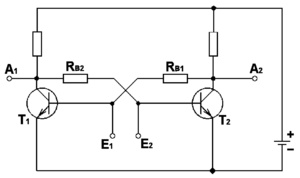A traditional latch circuit based on bipolar junction transistors

Flip-flops can be either simple (transparent or asynchronous) or clocked (synchronous); the transparent ones are commonly called latches. The word latch is mainly used for storage elements, while clocked devices are described as flip-flops.

Simple flip-flops can be built around a pair of cross-coupled inverting elements: vacuum tubes, bipolar transistors, field effect transistors, inverters, and inverting logic gates have all been used in practical circuits. Clocked devices are specially designed for synchronous systems; such devices ignore their inputs except at the transition of a dedicated clock signal (known as clocking, pulsing, or strobing). Clocking causes the flip-flop to either change or retain its output signal based upon the values of the input signals at the transition. Some flip-flops change output on the rising edge of the clock, others on the falling edge.

Since the elementary amplifying stages are inverting, two stages can be connected in succession (as a cascade) to form the needed non-inverting amplifier. In this configuration, each amplifier may be considered as an active inverting feedback network for the other inverting amplifier. Thus the two stages are connected in a non-inverting loop although the circuit diagram is usually drawn as a symmetric cross-coupled pair (both the drawings are initially introduced in the Eccles–Jordan patent).

## Flip-flop types

Flip-flops can be divided into common types: the SR ("set-reset"), D ("data" or "delay"), T ("toggle"), and JK types are the common ones. The behavior of a particular type can be described by what is termed the characteristic equation, which derives the "next" (i.e., after the next clock pulse) output, Qnext, in terms of the input signal(s) and/or the current output, Q.

### Simple set-reset latches

#### SR NOR latchAn SR latch, constructed from a pair of cross-coupled NOR gates (an animated picture). Red and black mean logical '1' and '0', respectively.

When using static gates as building blocks, the most fundamental latch is the simple SR latch, where S and R stand for set and reset. It can be constructed from a pair of cross-coupled NOR logic gates. The stored bit is present on the output marked Q.

While the S and R inputs are both low, feedback maintains the Q and Q outputs in a constant state, with Q the complement of Q. If S (Set) is pulsed high while R (Reset) is held low, then the Q output is forced high, and stays high when S returns to low; similarly, if R is pulsed high while S is held low, then the Q output is forced low, and stays low when R returns to low.

SR latch operation
S R Action
0 0 No Change
0 1 Q = 0
1 0 Q = 1
1 1 Restricted combination

The R = S = 1 combination is called a restricted combination or a forbidden state because, as both NOR gates then output zeros, it breaks the logical equation Q = not Q. The combination is also inappropriate in circuits where both inputs may go low simultaneously (i.e. a transition from restricted to keep). The output would lock at either 1 or 0 depending on the propagation time relations between the gates (a race condition). In certain implementations, it could also lead to longer ringings (damped oscillations) before the output settles, and thereby result in undetermined values (errors) in high-frequency digital circuits. Although this condition is usually avoided, it can be useful in some applications.

To overcome the restricted combination, one can add gates to the inputs that would convert (S,R) = (1,1) to one of the non-restricted combinations. That can be:

• Q = 1 (1,0) – referred to as an S-latch
• Q = 0 (0,1) – referred to as an R-latch
• Keep state (0,0) – referred to as an E-latch

Alternatively, the restricted combination can be made to toggle the output. The result is the JK latch.

Characteristic: Q+ = R'Q + R'S or Q+ = R'Q + S.

#### SR NAND latchAn SR latch

This is an alternate model of the simple SR latch built with NAND (not AND) logic gates. Set and reset now become active low signals, denoted S and R respectively. Otherwise, operation is identical to that of the SR latch. Historically, SR-latches have been predominant despite the notational inconvenience of active-low inputs.[citation needed]

SR latch operation
S R Action
0 0 Restricted combination
0 1 Q = 1
1 0 Q = 0
1 1 No ChangeSymbol for an SR NAND latch

#### JK latch

The JK latch is much less used than the JK flip-flop. The JK latch follows the following state table:

 JK latch truth table J K Qnext Comment 0 0 Q No change 0 1 0 Reset 1 0 1 Set 1 1 Q Toggle

Hence, the JK latch is an SR latch that is made to toggle its output when passed the restricted combination of 11. Unlike the JK flip-flop, the 11 input combination for the JK latch is not useful because there is no clock that directs toggling.

### Gated latches and conditional transparency

Latches are designed to be transparent. That is, input signal changes cause immediate changes in output; when several transparent latches follow each other, using the same clock signal, signals can propagate through all of them at once. Alternatively, additional logic can be added to a simple transparent latch to make it non-transparent or opaque when another input (an "enable" input) is not asserted. By following a transparent-high latch with a transparent-low (or opaque-high) latch, a master–slave flip-flop is implemented.

#### Gated SR latch

A synchronous SR latch (sometimes clocked SR flip-flop) can be made by adding a second level of NAND gates to the inverted SR latch (or a second level of AND gates to the direct SR latch). The extra gates further invert the inputs so the simple SR latch becomes a gated SR latch (and a simple SR latch would transform into a gated SR latch with inverted enable).

With E high (enable true), the signals can pass through the input gates to the encapsulated latch; all signal combinations except for (0,0) = hold then immediately reproduce on the (Q,Q) output, i.e. the latch is transparent.

With E low (enable false) the latch is closed (opaque) and remains in the state it was left the last time E was high.

The enable input is sometimes a clock signal, but more often a read or write strobe.

Gated SR latch operation
E/C Action
0 No action (keep state)
1 The same as non-clocked SR latch

#### Gated D latch

This latch exploits the fact that in the two active input combinations (01 and 10) of a gated SR latch R is the complement of S. The input NAND stage converts the two D input states (0 and 1) to these two input combinations for the next SR latch by inverting the data input signal. The low state of the enable signal produces the inactive "11" combination. Thus a gated D-latch may be considered as a one-input synchronous SR latch. This configuration prevents from applying the restricted combination to the inputs. It is also known as transparent latch, data latch, or simply gated latch. It has a data input and an enable signal (sometimes named clock, or control). The word transparent comes from the fact that, when the enable input is on, the signal propagates directly through the circuit, from the input D to the output Q.

Transparent latches are typically used as I/O ports or in asynchronous systems, or in synchronous two-phase systems (synchronous systems that use a two-phase clock), where two latches operating on different clock phases prevent data transparency as in a master–slave flip-flop.

Latches are available as integrated circuits, usually with multiple latches per chip. For example, 74HC75 is a quadruple transparent latch in the 7400 series.

E/C D Q Q Comment 0 X Qprev Qprev No change 1 0 0 1 Reset 1 1 1 0 Set

The truth table shows that when the enable/clock input is 0, the D input has no effect on the output. When E/C is high, the output equals D.

#### Earle latchEarle latch uses complementary Enable inputs: Enable active Low (E_L) and Enable active H (E_H)

The classic gated latch designs have some undesirable characteristics. They require double-rail logic or an inverter. The input-to-output propagation may take up to three gate delays. The input-to-output propagation is not constant – some outputs take two gate delays while others take three.

Designers looked for alternatives. A successful alternative is the Earle latch. It requires only a single data input, and its output takes a constant two gate delays. In addition, the two gate levels of the Earle latch can be merged with the last two gate levels of the circuits driving the latch. Merging the latch function can implement the latch with no additional gate delays.

The Earle latch is hazard free. If the middle NAND gate is omitted, then one gets the polarity hold latch, which is commonly used because it demands less logic. Intentionally skewing the clock signal can avoid the hazard.

### D flip-flop

The D ﬂip-ﬂop is the most common flip-flop in use today. It is better known as data or delay flip-flop (as its output Q looks like a delay of input D).

The Q output takes on the state of the D input at the moment of a positive edge at the clock pin (or negative edge if the clock input is active low). It is called the D flip-flop for this reason, since the output takes the value of the D input or data input, and delays it by one clock cycle. The D flip-flop can be interpreted as a primitive memory cell, zero-order hold, or delay line. Whenever the clock pulses, the value of Qnext is D and Qprev otherwise.

Truth table:

 Clock D Q Qprev Rising edge 0 0 X Rising edge 1 1 X Non-Rising X Qprev

('X' denotes a Don't care condition, meaning the signal is irrelevant)

Most D-type flip-flops in ICs have the capability to be forced to the set or reset state (which ignores the D and clock inputs), much like an SR flip-flop. Usually, the illegal S = R = 1 condition is resolved in D-type flip-flops. By setting S = R = 0, the flip-flop can be used as described above.

Inputs Outputs
S R D > Q Q'
0 1 X X 0 1
1 0 X X 1 0
1 1 X X 1 1

These flip-flops are very useful, as they form the basis for shift registers, which are an essential part of many electronic devices. The advantage of the D flip-flop over the D-type "transparent latch" is that the signal on the D input pin is captured the moment the flip-flop is clocked, and subsequent changes on the D input will be ignored until the next clock event. An exception is that some flip-flops have a "reset" signal input, which will reset Q (to zero), and may be either asynchronous or synchronous with the clock.

The above circuit shifts the contents of the register to the right, one bit position on each active transition of the clock. The input X is shifted into the leftmost bit position.

#### Classical positive-edge-triggered D flip-flop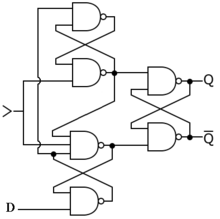A positive-edge-triggered D flip-flop

This clever circuit consists of two stages implemented by SR NAND latches. The input stage (the two latches on the left) processes the clock and data signals to ensure correct input signals for the output stage (the single latch on the right). If the clock is low, both the output signals of the input stage are high regardless of the data input; the output latch is unaffected and it stores the previous state. When the clock signal changes from low to high, only one of the output voltages (depending on the data signal) goes low and sets/resets the output latch: if D = 0, the lower output becomes low; if D = 1, the upper output becomes low. If the clock signal continues staying high, the outputs keep their states regardless of the data input and force the output latch to stay in the corresponding state as the input logical zero remains active while the clock is high. Hence the role of the output latch is to store the data only while the clock is low.

The circuit is closely related to the gated D latch as both the circuits convert the two D input states (0 and 1) to two input combinations (01 and 10) for the output SR latch by inverting the data input signal (both the circuits split the single D signal in two complementary S and R signals). The difference is that in the gated D latch simple NAND logical gates are used while in the positive-edge-triggered D flip-flop SR NAND latches are used for this purpose. The role of these latches is to "lock" the active output producing low voltage (a logical zero); thus the positive-edge-triggered D flip-flop can be thought of as a gated D latch with latched input gates.

#### Master–slave pulse-triggered D flip-flop

A master–slave D flip-flop is created by connecting two gated D latches in series, and inverting the enable input to one of them. It is called master–slave because the second latch in the series only changes in response to a change in the first (master) latch.

The term pulse-triggered means that data is entered on the rising edge of the clock pulse, but the output does not reflect the change until the falling edge of the clock pulse.[dubious ]A master–slave D flip-flop. It responds on the negative edge of the enable input (usually a clock)An implementation of a master–slave D flip-flop that is triggered on the positive edge of the clock

For a positive-edge triggered master–slave D flip-flop, when the clock signal is low (logical 0) the "enable" seen by the first or "master" D latch (the inverted clock signal) is high (logical 1). This allows the "master" latch to store the input value when the clock signal transitions from low to high. As the clock signal goes high (0 to 1) the inverted "enable" of the first latch goes low (1 to 0) and the value seen at the input to the master latch is "locked". Nearly simultaneously, the twice inverted "enable" of the second or "slave" D latch transitions from low to high (0 to 1) with the clock signal. This allows the signal captured at the rising edge of the clock by the now "locked" master latch to pass through the "slave" latch. When the clock signal returns to low (1 to 0), the output of the "slave" latch is "locked", and the value seen at the last rising edge of the clock is held while the "master" latch begins to accept new values in preparation for the next rising clock edge.

By removing the leftmost inverter in the circuit at side, a D-type flip flop that strobes on the falling edge of a clock signal can be obtained. This has a truth table like this:

 D Q > Qnext 0 X Falling 0 1 X Falling 1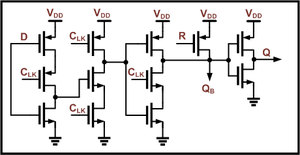A CMOS IC implementation of a "true single-phase edge-triggered flip-flop with reset"

#### Edge-triggered dynamic D storage element

An efficient functional alternative to a D flip-flop can be made with dynamic circuits as long as it is clocked often enough; while not a true flip-flop, it is still called a flip-flop for its functional role. While the master–slave D element is triggered on the edge of a clock, its components are each triggered by clock levels. The "edge-triggered D flip-flop", as it is called even though it is not a true flip-flop, does not have the master–slave properties.

Edge-triggered D flip-flops are often implemented in integrated high-speed operations using dynamic logic. This means that the digital output is stored on parasitic device capacitance while the device is not transitioning. This design of dynamic flip flops also enables simple resetting since the reset operation can be performed by simply discharging one or more internal nodes. A common dynamic flip-flop variety is the true single-phase clock (TSPC) type which performs the flip-flop operation with little power and at high speeds. However, dynamic flip-flops will typically not work at static or low clock speeds: given enough time, leakage paths may discharge the parasitic capacitance enough to cause the flip-flop to enter invalid states.

### T flip-flop

If the T input is high, the T flip-flop changes state ("toggles") whenever the clock input is strobed. If the T input is low, the flip-flop holds the previous value. This behavior is described by the characteristic equation:$Q_{next} = T \oplus Q = T\overline{Q} + \overline{T}Q$ (expanding the XOR operator)

and can be described in a truth table:

T flip-flop operation
Characteristic table Excitation table
T Q Qnext Comment Q Qnext T Comment
0 0 0 hold state (no clk) 0 0 0 No change
0 1 1 hold state (no clk) 1 1 0 No change
1 0 1 toggle 0 1 1 Complement
1 1 0 toggle 1 0 1 Complement

When T is held high, the toggle flip-flop divides the clock frequency by two; that is, if clock frequency is 4 MHz, the output frequency obtained from the flip-flop will be 2 MHz. This "divide by" feature has application in various types of digital counters. A T flip-flop can also be built using a JK flip-flop (J & K pins are connected together and act as T) or D flip-flop (T input and Qprevious is connected to the D input through an XOR gate). A T flip-flop can also be built using an edge-triggered D flip-flop with its D input fed from its own inverted output.

### JK flip-flop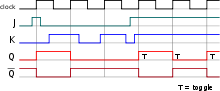JK flip-flop timing diagram

The JK flip-flop augments the behavior of the SR flip-flop (J=Set, K=Reset) by interpreting the S = R = 1 condition as a "flip" or toggle command. Specifically, the combination J = 1, K = 0 is a command to set the flip-flop; the combination J = 0, K = 1 is a command to reset the flip-flop; and the combination J = K = 1 is a command to toggle the flip-flop, i.e., change its output to the logical complement of its current value. Setting J = K = 0 does NOT result in a D flip-flop, but rather, will hold the current state. To synthesize a D flip-flop, simply set K equal to the complement of J. The JK flip-flop is therefore a universal flip-flop, because it can be configured to work as an SR flip-flop, a D flip-flop, or a T flip-flop.

The characteristic equation of the JK flip-flop is:$Q_{next} = J\overline Q + \overline KQ$

and the corresponding truth table is:

JK Flip Flop operation
Characteristic table Excitation table
J K Qnext Comment Q Qnext J K Comment
0 0 Q hold state 0 0 0 X No change
0 1 0 reset 0 1 1 X Set
1 0 1 set 1 0 X 1 Reset
1 1 Q toggle 1 1 X 0 No change

## Metastability

Flip-flops are prone to a problem called metastability, which can happen when two inputs, such as data and clock or clock and reset, are changing at about the same time, such that the resulting state would depend on the order of the input events. When the order is not clear, within appropriate timing constraints, the result is that the output may behave unpredictably, taking many times longer than normal to settle to one state or the other, or even oscillating several times before settling. Theoretically, the time to settle down is not bounded. In a computer system, this metastability can cause corruption of data or a program crash, if the state is not stable before another circuit uses its value; in particular, if two different logical paths use the output of a flip-flop, one path can interpret it as a 0 and the other as a 1 when it has not resolved to stable state, putting the machine into an inconsistent state.

## Timing considerations

### Setup and hold timesFlip-flop setup, hold and clock-to-output timing parameters

Setup time is the minimum amount of time the data signal should be held steady before the clock event so that the data are reliably sampled by the clock. This applies to synchronous circuits such as the flip-flop.

Hold time is the minimum amount of time the data signal should be held steady after the clock event so that the data are reliably sampled. This applies to synchronous circuits such as the flip-flop.

To summarize: Setup time -> Clock flank -> Hold time.

The metastability in flip-flops can be avoided by ensuring that the data and control inputs are held valid and constant for specified periods before and after the clock pulse, called the setup time (tsu) and the hold time (th) respectively. These times are specified in the data sheet for the device, and are typically between a few nanoseconds and a few hundred picoseconds for modern devices.

Unfortunately, it is not always possible to meet the setup and hold criteria, because the flip-flop may be connected to a real-time signal that could change at any time, outside the control of the designer. In this case, the best the designer can do is to reduce the probability of error to a certain level, depending on the required reliability of the circuit. One technique for suppressing metastability is to connect two or more flip-flops in a chain, so that the output of each one feeds the data input of the next, and all devices share a common clock. With this method, the probability of a metastable event can be reduced to a negligible value, but never to zero. The probability of metastability gets closer and closer to zero as the number of flip-flops connected in series is increased.

So-called metastable-hardened flip-flops are available, which work by reducing the setup and hold times as much as possible, but even these cannot eliminate the problem entirely. This is because metastability is more than simply a matter of circuit design. When the transitions in the clock and the data are close together in time, the flip-flop is forced to decide which event happened first. However fast we make the device, there is always the possibility that the input events will be so close together that it cannot detect which one happened first. It is therefore logically impossible to build a perfectly metastable-proof flip-flop.

### Propagation delay

Another important timing value for a flip-flop (F/F) is the clock-to-output delay (common symbol in data sheets: tCO) or propagation delay (tP), which is the time the flip-flop takes to change its output after the clock edge. The time for a high-to-low transition (tPHL) is sometimes different from the time for a low-to-high transition (tPLH).

When cascading F/Fs which share the same clock (as in a shift register), it is important to ensure that the tCO of a preceding F/F is longer than the hold time (th) of the following flip-flop, so data present at the input of the succeeding F/F is properly "shifted in" following the active edge of the clock. This relationship between tCO and th is normally guaranteed if the F/Fs are physically identical. Furthermore, for correct operation, it is easy to verify that the clock period has to be greater than the sum tsu + th.

## Generalizations

Flip-flops can be generalized in at least two ways: by making them 1-of-N instead of 1-of-2, and by adapting them to logic with more than two states. In the special cases of 1-of-3 encoding, or multi-valued ternary logic, these elements may be referred to as flip-flap-flops.

In a conventional flip-flop, exactly one of the two complementary outputs is high. This can be generalized to a memory element with N outputs, exactly one of which is high (alternatively, where exactly one of N is low). The output is therefore always a one-hot (respectively one-cold) representation. The construction is similar to a conventional cross-coupled flip-flop; each output, when high, inhibits all the other outputs. Alternatively, more or less conventional flip-flops can be used, one per output, with additional circuitry to make sure only one at a time can be true.

Another generalization of the conventional flip-flop is a memory element for multi-valued logic. In this case the memory element retains exactly one of the logic states until the control inputs induce a change. In addition, a multiple-valued clock can also be used, leading to new possible clock transitions.

Wikimedia Foundation. 2010.

Поможем написать реферат

### Look at other dictionaries:

• Flip-flop (disambiguation) — A flip flop is a type of sandal normally worn at beaches, often made with a foam rubber sole and two rubber straps that go between the middle side of the outer edge of the sole and the big toe to second toe gap.Flip flop may also refer to:* Flip… …   Wikipedia

• flip-flop — /flip flop /, n., adv., v., flip flopped, flip flopping. n. 1. Informal. a sudden or unexpected reversal, as of direction, belief, attitude, or policy. 2. a backward somersault. 3. Also called flip flop circuit. Electronics. an electronic circuit …   Universalium

• flip-flop — [flip′fläp΄] n. ☆ 1. an acrobatic spring backward from the feet to the hands and back to the feet 2. an abrupt change, as to an opposite opinion 3. a flapping noise 4. THONG (sense 3) 5. Electronics a circuit having two stable states and… …   English World dictionary

• flip-flop — || flÉªpâ€šflÉ‘p /flÉ’p (Informal) abrupt reversal or change (esp. of opinion); back flip; sound of something flapping; backless thong sandal; circuit that can toggle between two modes (Electronics) …   English contemporary dictionary

• flip-flop — ► NOUN 1) a light sandal with a thong that passes between the big and second toes. 2) Electronics a switching circuit which works by changing between two stable states. ► VERB ▪ move with a flapping sound or motion …   English terms dictionary

• flip-flop — noun 1》 a light sandal with a thong that passes between the big and second toes. 2》 N. Amer. a backward somersault or handspring. 3》 N. Amer. informal an abrupt reversal of policy. 4》 Electronics a switching circuit which works by changing… …   English new terms dictionary

• Flop — may refer to: *Flop, a slang term for failure *Flop, a box office bomb in the entertainment world *Flop (poker), a poker term describing the first three cards dealt to the board *Flip flop (electronics), the bistable multivibrator, a circuit with …   Wikipedia

• electronics — I (New American Roget s College Thesaurus) Science of electrons Nouns 1. electronics, electron physics, electronic engineering, solid state electronics; musical instrument digital interface, MIDI; electron theory of atoms or solids;… …   English dictionary for students

• Metastability in electronics — For other uses of the term, see Metastability. Metastability in electronics is the ability of a digital electronic system to persist for an unbounded time in an unstable equilibrium or metastable state. In metastable states, the circuit may be …   Wikipedia

• Latch (electronics) — In electronics, a latch is a kind of bistable multivibrator, an electronic circuit which has two stable states and thereby can store one bit of information. Today the word is mainly used for simple transparent storage elements, while slightly… …   Wikipedia